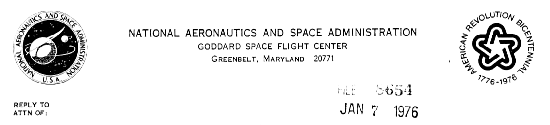TO: Don York, Ted Snow and Users of Copernicus Data at GSFC FROM: W. Budich, R. Bohlin and J. Drake SUBJECT: Corrections to the U2 Wavelength Scale Between 1026 and 1110Å.

To determine the correction to the U2 wavelengths several interstellar lines of molecular hydrogen between 1040Å and 1113Å were investigated in the spectra of 17 stars. The position of the lines were measured in the frame of the most prominent interstellar feature in the visible. Deviations of the H2 velocities from the visible value and any other random shifts were assumed to average to zero for the 17 stars as a group. The average shifts were plotted and approximated by the two straight lines shown in Figure 1. The fit was drawn through the (1026, 0.18Å) point found earlier by Bohlin during a study of Lyman Beta. In the course of the Lyman Alpha survey work, no evidence for a systematic shift at 1216Å was found. The U2 wavelength scale at La is certainly well within the quoted error of ±0.05Å. The equations for the two straight lines are

Delta Lambda = -0.002425Lambda + 2.668 for Lambda < 1070Å

and

Delta Lambda = -0.00066Lambda + 0.779 for Lambda > 1070Å.

In the implementation of the shifts in the GSFC reduction, the equations are applied for all Lambda < 1180Å, where the correction is zero.

Table 1 shows the residuals between the actual and measured wavelengths after applying the above correction. The averages shown for each star are the combination of the actual difference between the H2 and visual cloud velocities, random errors in the procedure, and temperature or other instrumentally caused shifts. The average residual shifts are applied to the data before attempting to derive H2 column densities.

/Ralph/
Ralph C. Bohlin
Observational Astronomy Branch